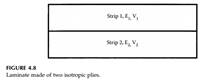### Create an Account

Already have account?

### Forgot Your Password ?

Home / Questions / Give expressions for the stiffness matrices A B and D for an isotropic material in terms...

# Give expressions for the stiffness matrices A B and D for an isotropic material in terms of its thickness t Youngs modulus E and Poissons ratio ν 2 Show that for a symmetric

Give expressions for the stiffness matrices [A], [B], and [D] for an isotropic material in terms of its thickness, t, Young’s modulus, E, and Poisson’s ratio, ν.

2. Show that, for a symmetric laminate, the coupling stiffness matrix is equal to zero.

3. A beam is made of two bonded isotropic strips as shown in the Figure 4.8. The two strips are of equal thickness. Find the stiffness matrices [A], [B], and [D].Jun 28 2020 View more View Less

#### Answer (Solved)Subscribe To Get Solution